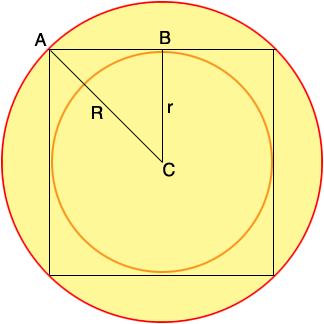SEARCH HOMEMath Central Quandaries & QueriesQuestion from jouniella, a student: A square is inscribe to the first circle, then another circle is inscribe to the square. Find the ratio of the 2 circles.Hi Jouniella,

I expect you mean "Find the ratio of the areas of the 2 circles."

Let R be the radius of the first circle and r the radius of the second, as indicated in the diagram.Write an expression for the area of each circle.

What does the symmetry of the circle tell you about the length of the line segment BA?

What does Pythagoras theorem tell you about the relationship between R2 and r2?

What is the ratio of the areas of the two circles?

PennyMath Central is supported by the University of Regina and The Pacific Institute for the Mathematical Sciences.